# Testing the periodic $G$ claim¶

In Anderson et al they claim to see a periodicity of 5.9 years in the CODATA measurements of Newton's gravitational constant $G$, which is consistent with the period of Length Of Day changes.

This claim can be tested through Bayesian model selection. I compute the Bayesian evidence for six hypothesis and compare them:

1. $H_1$ - the data variation can be described by Gaussian noise given by the experimental errors;
2. $H_2$ - the data variation can be described by Gaussian noise given by the experimental errors and an unknown systematic noise term;
3. $H_3$ - the data variation can be described by Gaussian noise given by the experimental errors, and a sinusoid with period 5.9 years, but with unknown amplitude and phase;
4. $H_4$ - the data variation can be described by Gaussian noise given by the experimental errors, an unknown systematic noise term, and a sinusoid with period 5.9 years, but with unknown amplitude and phase;
5. $H_5$ - the data variation can be described by Gaussian noise given by the experimental errors, an unknown systematic noise term, and a sinusoid with unknown period, phase and amplitude

In each case where I use a likelihood defined by $p(d|\vec{\theta},H,I) = (2\pi)^{-N/2}\left(\prod_{j=1}^N (\sigma_j^2 + \sigma_{\rm sys}^2)^{-1/2} \right) \exp{\left(-\sum_{j=1}^N \frac{(d_j - m_{j}(A, P, \phi_0))^2}{2(\sigma_j^2 + \sigma_{\rm sys}^2)} \right)}$ where $\sigma_{\rm sys}$ is a potentially unknown systematic noise term. In this case $\vec{\theta} =\{\sigma_{\rm sys}, A, P, \phi_0, t_0\}$.

The general model I use is $m_i(A, P, \phi_0, t_0) = A\sin{(\phi_0 + 2\pi (t_i-t_0)/P)} + \mu_G$ where $A$ is the sinusoid amplitude, $P$ is the period, $\phi_0$ is the initial phase, $t_0$ is the initial epoch, and I use the mean value of the data from Anderson et al of $\mu_G = 6.673899\times 10^{-11}$. In general $\phi_0$ and $t_0$ will be completely correlated, so I will just search over $\phi_0$ and have $t_0$ fixed at zero.

In :
%matplotlib inline
import matplotlib.pyplot as pl
import numpy as np

# set plots to use LaTeX for axes
pl.rc('text', usetex=True)
pl.rc('font', family='serif')
pl.rc('font', size=14)

# define the log likelihood function
def logL(data, model, sigmas, sigmasys):
N = len(data)
norm = -0.5*N*np.log(2.*np.pi) - np.sum(0.5*np.log(sigmas**2 + sigmasys**2))
chi2 = -0.5*np.sum((data-model)**2/(sigmas**2 + sigmasys**2))
return norm + chi2

# define the model function
def pmodel(A, P, phi0, t0, ts):
muG = 6.673899e-11
return A*np.sin(phi0 + 2.*np.pi*(ts-t0)/P) + muG


The data we use are (note some dates are publication, or manuscript received dates, and may not represent the actual experiment data, which are going to be earlier) (see also page 41 of Mohr, Taylor & Newell, which are generally the values I have used):

Ref. Date Value ($10^{-11} {\rm m}^3 {\rm sec}^{-2} {\rm kg}^{-1}$)
Luther & Towler 17 Nov 1982 (received) $6.67248 \pm 0.00043$
Bagley & Luther 10 Dec 1996 (received) $6.67398 \pm 0.00070$
Kleinevoss et al 20 Jan 1999 (received) $6.6735\pm 0.0011 \pm 0.0026$
Gundlach & Merkowitz 5 Jun 2000 (received) $6.674255 \pm 0.000092$
Quinn et al 12 Feb 2001 (received) $6.67559\pm 0.00027$
Armstrong & Fitzgerald 12 May 2003 (received) $6.67387\pm0.00027$
Hu, Guo & Luo 17 Apr 1998 (received from previous paper) $6.67228\pm0.00087$
Schlamminger et al 12 Jun 2006 (received) $6.674252\pm0.000120$
Luo et al 2 Mar 2009 (revisions received1) $6.67349\pm0.00018$
Parks & Faller 01 Jun 2004 (data collected in 2004) $6.67234\pm0.00014$
Rosi et al 15 Jul 2013 (data collected) $6.67191\pm0.00099$

I exclude the Karagioz & Izmailov results from 1996 as they are an average of previous 10 years of results. I also exclude The Kleinevoss (2002) reults as I don't know the date, but do add an earlier Kleinevoss result that has quite large uncertainties. I also exclude the Quinn et al results (see also erratum) as they cover a period of 4 years between 2004 to 2008.

Note: as the dates are mainly submitted dates the actual times of the experimental results may be of order several months earlier, so a proper analysis might include errors on these (or the experimenters could be contacted for the actual dates!)

1 I assume new measurements were taken after the initial submission date of 7 May 2006, so use the later revision date.

In :
import calendar
import datetime

# the data (dates, values of G )
dates =          ["17Nov1982", "10Dec1996", "20Jan1999",                        "05Jun2000",  "12Feb2001", "12May2003", "17Apr1998", "12Jun2006",  "02Mar2009", "01Jun2004", "15Jul2013"]
Gs =    np.array([6.67248e-11, 6.67398e-11, 6.6735e-11,                         6.674255e-11, 6.67559e-11, 6.67387e-11, 6.67228e-11, 6.674252e-11, 6.67349e-11, 6.67234e-11, 6.67191e-11])
errs =  np.array([0.00043e-11, 0.00070e-11, np.sqrt(0.0011**2+0.0026**2)*1e-11, 0.000092e-11, 0.00027e-11, 0.00027e-11, 0.00087e-11, 0.000120e-11, 0.00018e-11, 0.00014e-11, 0.00099e-11])

datestr = [datetime.datetime.strptime(d, "%d%b%Y") for d in dates]
utctime = [calendar.timegm(d.utctimetuple()) for d in datestr]

# convert times to years
years =[(d-utctime)/(86400.*365.25) for d in utctime]
years = np.array(years)

pl.errorbar(years, Gs, yerr=errs, fmt='bo')
pl.xlabel('time (years from 17 Nov 1982)')
pl.ylabel(r'$G \mathrm{m}^3\,\mathrm{s}^{-2}\,\mathrm{kg}^{-1}$')

Out:
<matplotlib.text.Text at 0x7f9f2f0389d0>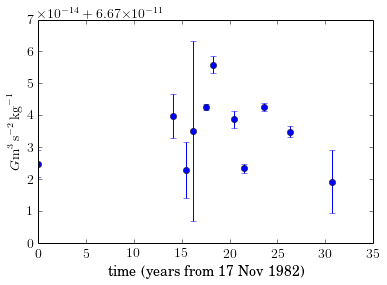## Case 1¶

Here I calculate the natural logarithm of the evidence, $Z_1$, for hypothesis 1: the data variation can be described by Gaussian noise given by the experimental errors. This is just performed by evaluating the likelihood function: $Z_1 = \ln{\left(p(d|\{\sigma_{\rm sys}=0, A=0, P=1, \phi_0=0, t_0=0\},H_1,I)\right)}$ Note: I use a value of $P=1$ to stop the model having to divide by zero, although the sinusoidal component will still be zero.

In :
model = pmodel(0., 1., 0., 0., 0.)
Z1 = logL(Gs, model, errs, 0.)
print Z1

250.38113464



## Case 2¶

Here I calculate the evidence, $Z_2$, for hypothesis 2: the data variation can be described by Gaussian noise given by the experimental errors and an unknown systematic noise term.

The unknown noise term will have a Jeffrey's prior of $p(\sigma_{\rm sys}|I) = \left(\ln{\left( \frac{\sigma_{\rm sys,max}}{\sigma_{\rm sys,min}} \right)\sigma_{\rm sys} }\right)^{-1}$ where I have set the limits of $\sigma_{\rm sys}$ using the smallest measured error as a lower limit and the maximum range of values as an upper limit.

The evidence has been evaluated as $Z_2 = \ln{\left( \int_{\sigma_{\rm sys,min}}^{\sigma_{\rm sys,max}} p(d|\{\sigma_{\rm sys}, A=0, P=1, \phi_0=0, t_0=0\},H_2,I)p(\sigma_{\rm sys}|I) {\rm d}\sigma_{\rm sys} \right)}$

In :
from scipy.misc import logsumexp

# define a trapezium rule integration function for log values
def logtrapz(arr, dx):
logt = -np.inf
ldx = np.log(dx)
l2 = np.log(2.)
for i in range(1,len(arr)):

return logt

sigmasysmax = np.max(Gs)-np.min(Gs)
sigmasysmin = np.min(errs)

sysfrac = np.log(sigmasysmax/sigmasysmin)

sysrange = np.linspace(sigmasysmin, sigmasysmax, 30)

# log prior for systematic error term
def logpriorsys(sys):
return -np.log(sysfrac)-np.log(sys)

Lpost = np.zeros(len(sysrange))
model = pmodel(0., 1., 0., 0., 0.)
for i in range(len(sysrange)):
Lpost[i] = logL(Gs, model, errs, sysrange[i])
Lpost[i] = Lpost[i] + logpriorsys(sysrange[i])

fig = pl.figure(figsize=(6,5), dpi=200)

pl.plot(sysrange, np.exp(Lpost-np.max(Lpost)))
pl.xlabel(r'$\sigma_{\mathrm{sys}}$', fontsize=16)
pl.ylabel(r'$p(\sigma_{\mathrm{sys}}|d,H_2,I)$', fontsize=16)

# marginalise over sysrange
Z2 = logtrapz(Lpost, np.diff(sysrange))
print Z2

335.342774121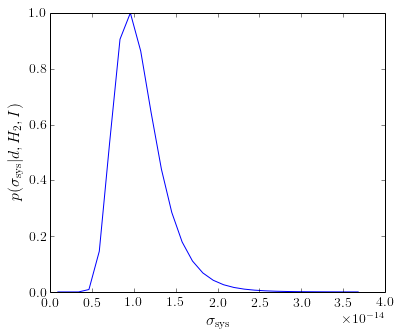## Case 3¶

Here I calculate the evidence, $Z_3$, for hypothesis 3: the data variation can be described by Gaussian noise given by the experimental errors, and a sinusoid with period 5.9 years, but with unknown amplitude and phase.

For the unknown sinusoid amplitude I use the same Jeffreys prior as for the unknown noise term $p(A|I) = \left(\ln{\left( \frac{A_{\rm max}}{A_{\rm min}} \right)A }\right)^{-1},$ with the upper and lower ranges $A_{\rm max}$ and $A_{\rm min}$ set to the same values as those for $\sigma_{\rm sys}$.

For the unknown phase I use a uniform prior between 0 and $2\pi$, giving $p(\phi_0|I) = \frac{1}{2\pi}.$

The evidence has been evaluated as $Z_3 = \ln{\left( \int_{A_{\rm min}}^{A_{\rm max}} \int_{\phi_{0,{\rm min}}}^{\phi_{0,{\rm max}}} p(d|\{\sigma_{\rm sys}=0, A, P=5.9, \phi_0, t_0=0\},H_3,I) p(A|I) p(\phi_0|I) {\rm d}A{\rm d}\phi_0) \right)}$

In :
# unknown sinusoid amplitude
Amax = np.max(Gs)-np.min(Gs)
Amin = np.min(errs)

Afrac = sysfrac

Arange = np.linspace(Amin, Amax, 30)

# log prior for sinusoid amplitude term
def logpriorA(A):
return -np.log(Afrac)-np.log(A)

# sinusoid phase
phi0max = 2.*np.pi
phi0min = 0.

phi0range = np.linspace(phi0min, phi0max, 30)

# log prior for sinusoid initial phase
logpriorphi0 = -np.log(2.*np.pi)

# period
P = 5.9

Lpost = np.zeros((len(Arange), len(phi0range)))
for i in range(len(Arange)):
for j in range(len(phi0range)):
model = pmodel(Arange[i], P, phi0range[j], 0., years)
Lpost[i,j] = logL(Gs, model, errs, 0.)
Lpost[i,j] = Lpost[i,j] + logpriorA(Arange[i]) + logpriorphi0

# marginalise over parameters
marg1 = np.apply_along_axis(logtrapz, 1, Lpost, np.diff(phi0range))

Z3 = logtrapz(marg1, np.diff(Arange))
print Z3

# plot posteriors
f, ax = pl.subplots(1, 2, figsize=(10,6))

# plot amplitude posterior
ax.plot(sysrange, np.exp(marg1-Z3))
ax.set_xlabel('$A (\mathrm{m}^3\,\mathrm{s}^{-2}\,\mathrm{kg}^{-1})$')
ax.set_ylabel('$p(A|d,H_3,I)$')

# plot phi0 posterior
marg1 = np.apply_along_axis(logtrapz, 0, Lpost, np.diff(Arange))

ax.plot(phi0range, np.exp(marg1-Z3))
ax.set_xlabel('$\phi_0$ (rads)')
ax.set_ylabel('$p(\phi_0|d,H_3,I)$')
ax.set_xlim(0, 2.*np.pi)

327.161035066


Out:
(0, 6.283185307179586)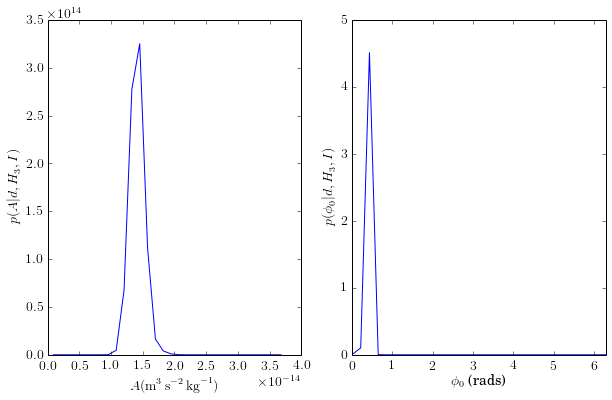## Case 4¶

Here I calculate the evidence, $Z_3$, for hypothesis 3: the data variation can be described by Gaussian noise given by the experimental errors, an unknown systematic noise term, and a sinusoid with period 5.9 years, but with unknown amplitude and phase.

For the amplitude and phase I use the same priors as for hypothesis 3 and for the unknown sytematic noise term I use the same prior as in hypothesis 2.

The evidence has been evaluated as $Z_4 = \ln{\left(\int_{\sigma_{\rm sys,min}}^{\sigma_{\rm sys,max}} \int_{A_{\rm min}}^{A_{\rm max}} \int_{\phi_{0,{\rm min}}}^{\phi_{0,{\rm max}}} p(d|\{\sigma_{\rm sys}, A, P=5.9, \phi_0, t_0=0\},H_4,I) p(\sigma_{\rm sys}|I)p(A|I) p(\phi_0|I) {\rm d}\sigma_{\rm sys}{\rm d}A{\rm d}\phi_0) \right)}$

In :
Lpost = np.zeros((len(sysrange), len(Arange), len(phi0range)))
for i in range(len(sysrange)):
for j in range(len(Arange)):
for k in range(len(phi0range)):
model = pmodel(Arange[j], P, phi0range[k], 0., years)
Lpost[i,j,k] = logL(Gs, model, errs, sysrange[i])
Lpost[i,j,k] = Lpost[i,j,k] + logpriorsys(sysrange[i]) + logpriorA(Arange[j]) + logpriorphi0

# marginalise over parameters
marg1 = np.apply_along_axis(logtrapz, 2, Lpost, np.diff(phi0range))
marg2 = np.apply_along_axis(logtrapz, 1, marg1, np.diff(Arange))

Z4 = logtrapz(marg2, np.diff(sysrange))
print Z4

# plot posteriors
f, ax = pl.subplots(3, figsize=(7,14))

# plot sigma_sys posterior
ax.plot(sysrange, np.exp(marg2-Z4))
ax.set_xlabel('$\sigma_{\mathrm{sys}} (\mathrm{m}^3\,\mathrm{s}^{-2}\,\mathrm{kg}^{-1})$')
ax.set_ylabel('$p(\sigma_{\mathrm{sys}}|d,H_4,I)$')

# plot amplitude posterior
marg1 = np.apply_along_axis(logtrapz, 2, Lpost, np.diff(phi0range))
marg2 = np.apply_along_axis(logtrapz, 0, marg1, np.diff(sysrange))

ax.plot(Arange, np.exp(marg2-Z4))
ax.set_xlabel('$A (\mathrm{m}^3\,\mathrm{s}^{-2}\,\mathrm{kg}^{-1})$')
ax.set_ylabel('$p(A|d,H_4,I)$')

# plot phi0 posterior
marg1 = np.apply_along_axis(logtrapz, 1, Lpost, np.diff(Arange))
marg2 = np.apply_along_axis(logtrapz, 0, marg1, np.diff(sysrange))

ax.plot(phi0range, np.exp(marg2-Z4))
ax.set_xlabel('$\phi_0$ (rads)')
ax.set_ylabel('$p(\phi_0|d,H_5,I)$')
ax.set_xlim(0, 2.*np.pi)

335.120556229


Out:
(0, 6.283185307179586)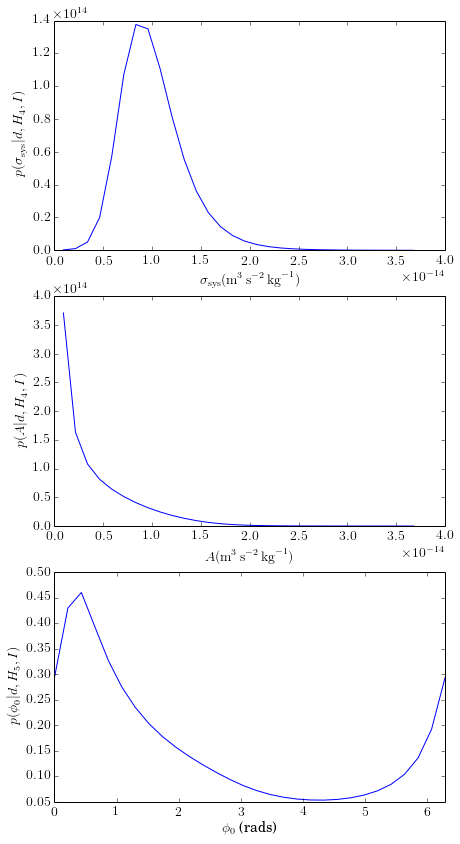## Case 5¶

Here I calculate the evidence, $Z_5$, for hypothesis 5: the data variation can be described by Gaussian noise given by the experimental errors, an unknown systematic noise term, and a sinusoid with unknown period, phase and amplitude.

For the phase, amplitude and systematic error terms I use the prior ranges defined for the previous hypotheses.

For the period I use a unform prior from 0.1 to the quasi-Nyquist frequency defined by the maximum time dfference between points (excluding the first point from 1982). As the epoch and phase will be completely correlated I only search over phase.

The evidence has been evaluated as $Z_5 = \ln{\left( \int_{\sigma_{\rm sys,min}}^{\sigma_{\rm sys,max}} \int_{A_{\rm min}}^{A_{\rm max}} \int_{\phi_{0,{\rm min}}}^{\phi_{0,{\rm max}}}\int_{P_{\rm min}}^{P_{\rm max}} p(d|\{\sigma_{\rm sys}, A, P, \phi_0, t_0=0\},H_5,I)p(\sigma_{\rm sys}|I)p(A|I)p(\phi_0|I)p(P|I) {\rm d}\sigma_{\rm sys}{\rm d}A{\rm d}\phi_0{\rm d}P \right)}$

In :
# period prior and range
timediff = np.diff(np.sort(years)[1:]) # skip the first longer time

nyq = 1./(2.*np.max(timediff))

periodmin = 0.1
periodmax = 1./nyq

logpriorperiod = -np.log(periodmax-periodmin)

periodrange = np.linspace(periodmin, periodmax, 40)

Lpost = np.zeros((len(sysrange), len(Arange), len(phi0range), len(periodrange)))
for i in range(len(sysrange)):
for j in range(len(Arange)):
for k in range(len(phi0range)):
for l in range(len(periodrange)):
model = pmodel(Arange[j], periodrange[l], phi0range[k], 0., years)
Lpost[i,j,k,l] = logL(Gs, model, errs, sysrange[i])
Lpost[i,j,k,l] = Lpost[i,j,k,l] + logpriorsys(sysrange[i]) + logpriorA(Arange[j]) + logpriorphi0 + logpriorperiod

# marginalise over parameters
marg1 = np.apply_along_axis(logtrapz, 3, Lpost, np.diff(periodrange))
marg2 = np.apply_along_axis(logtrapz, 2, marg1, np.diff(phi0range))
marg3 = np.apply_along_axis(logtrapz, 1, marg2, np.diff(Arange))

Z5 = logtrapz(marg3, np.diff(sysrange))
print Z5

# plot posteriors
f, ax = pl.subplots(2, 2, figsize=(10,10))

# plot sigma_sys posterior
ax[0,0].plot(sysrange, np.exp(marg3-Z5))
ax[0,0].set_xlabel('$\sigma_{\mathrm{sys}} (\mathrm{m}^3\,\mathrm{s}^{-2}\,\mathrm{kg}^{-1})$')
ax[0,0].set_ylabel('$p(\sigma_{\mathrm{sys}}|d,H_5,I)$')

# plot amplitude posterior
marg1 = np.apply_along_axis(logtrapz, 3, Lpost, np.diff(periodrange))
marg2 = np.apply_along_axis(logtrapz, 2, marg1, np.diff(phi0range))
marg3 = np.apply_along_axis(logtrapz, 0, marg2, np.diff(sysrange))

ax[0,1].plot(Arange, np.exp(marg3-Z5))
ax[0,1].set_xlabel('$A (\mathrm{m}^3\,\mathrm{s}^{-2}\,\mathrm{kg}^{-1})$')
ax[0,1].set_ylabel('$p(A|d,H_5,I)$')

# plot period posterior
marg1 = np.apply_along_axis(logtrapz, 2, Lpost, np.diff(Arange))
marg2 = np.apply_along_axis(logtrapz, 1, marg1, np.diff(phi0range))
marg3 = np.apply_along_axis(logtrapz, 0, marg2, np.diff(sysrange))

ax[1,0].plot(periodrange, np.exp(marg3-Z5))
ax[1,0].set_xlabel('$P$ (years)')
ax[1,0].set_ylabel('$p(P|d,H_5,I)$')
ax[1,0].set_xlim(periodrange, periodrange[-1])

# plot phi0 posterior
marg1 = np.apply_along_axis(logtrapz, 3, Lpost, np.diff(periodrange))
marg2 = np.apply_along_axis(logtrapz, 1, marg1, np.diff(Arange))
marg3 = np.apply_along_axis(logtrapz, 0, marg2, np.diff(sysrange))

ax[1,1].plot(phi0range, np.exp(marg3-Z5))
ax[1,1].set_xlabel('$\phi_0$ (rads)')
ax[1,1].set_ylabel('$p(\phi_0|d,H_5,I)$')
ax[1,1].set_xlim(0, 2.*np.pi)

# get marginal distribution for period and amplitude
marg1 = np.apply_along_axis(logtrapz, 2, Lpost, np.diff(phi0range))
marg2 = np.apply_along_axis(logtrapz, 0, marg1, np.diff(sysrange))

post2d = np.exp(marg2-Z5)
fig = pl.figure(figsize=(6,5))
pl.imshow(np.flipud(post2d), extent=[periodrange, periodrange[-1], Arange, Arange[-1]], aspect='auto')
pl.xlabel('$P$ (years)')
pl.ylabel('$A (\mathrm{m}^3\,\mathrm{s}^{-2}\,\mathrm{kg}^{-1})$')

335.685311193


Out:
<matplotlib.text.Text at 0x7f9f2d9d8e50>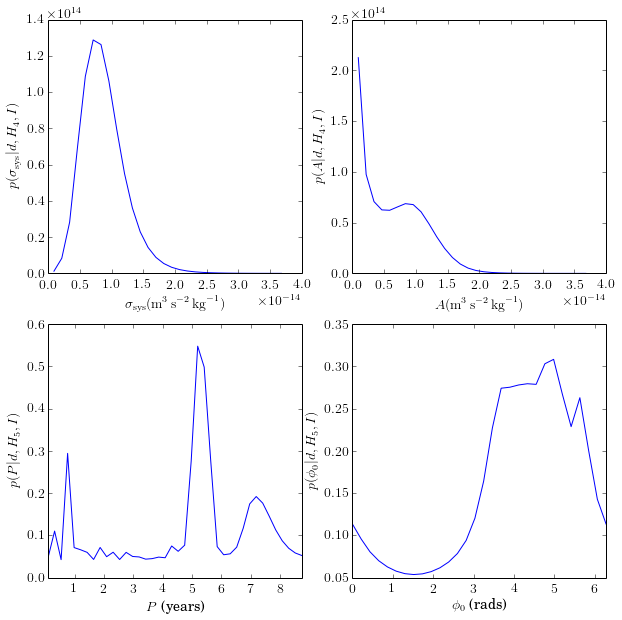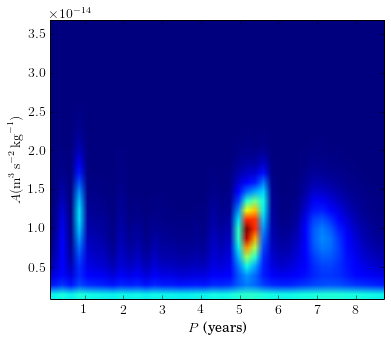Now get the model comparison results.

In :
# print out results
print("Z1 = %.1f\nZ2 = %.1f\nZ3 = %.1f\nZ4 = %.1f\nZ5 = %.1f" % (Z1, Z2, Z3, Z4, Z5))
print("exp(Z2-Z1) = exp(%.1f),\texp(Z3-Z1) = exp(%.1f),\texp(Z4-Z1) = exp(%.1f),\texp(Z5-Z1) = exp(%.1f)" % ((Z2-Z1), (Z3-Z1), (Z4-Z1), (Z5-Z1) ))
print("exp(Z3-Z2) = %.5f,\texp(Z4-22) = %.5f,\texp(Z5-Z2) = %.5f" % (np.exp(Z3-Z2), np.exp(Z4-Z2), np.exp(Z5-Z2)))
print("exp(Z4-Z3) = %.5f,\texp(Z5-Z3) = %.5f" % (np.exp(Z4-Z3), np.exp(Z5-Z3)))
print("exp(Z5-Z4) = %.5f" % (np.exp(Z5-Z4)))

Z1 = 250.4
Z2 = 335.3
Z3 = 327.2
Z4 = 335.1
Z5 = 335.7
exp(Z2-Z1) = exp(85.0),	exp(Z3-Z1) = exp(76.8),	exp(Z4-Z1) = exp(84.7),	exp(Z5-Z1) = exp(85.3)
exp(Z3-Z2) = 0.00028,	exp(Z4-22) = 0.80074,	exp(Z5-Z2) = 1.40852
exp(Z4-Z3) = 2862.70186,	exp(Z5-Z3) = 5035.54039
exp(Z5-Z4) = 1.75902



So, the final table of log evidences is

$Z_1$ $Z_2$ $Z_3$ $Z_4$ $Z_5$
$250.4$ $335.3$ $327.2$ $335.1$ $335.7$

Or, in terms of a table of odds ratios ($i$ are columns and $j$ are rows)

$\mathcal{O}_{ij} = \exp{\left(\frac{Z_i}{Z_j}\right)}$ $Z_2$ $Z_3$ $Z_4$ $Z_5$
$Z_1$ $e^{85.0}$ $e^{76.8}$ $e^{84.7}$ $e^{85.3}$
$Z_2$ $0.0003$ $0.8$ $1.4$
$Z_3$ $2862.7$ $5053.5$
$Z_4$ $1.8$

What I find is that all hypotheses that include additional components over the purely Gaussian experimental errors are hugely favoured over hypothesis 1 that just assumes experimental errors are enough to account for the variations.

The most favoured hypothesis is 5, which includes the possiblity of a sinusoidal term of unknown period, phase and amplitude, and an unknown systematic noise term. However, this is only favoured over hypothesis 2, which just includes the unknown systematic noise term, by a factor of 1.4, so is hardly significant.

It is interesting to look at the posterior parameter distribution obtained from hypothesis 5, which clearly does show some evidence for a periodic component, although it is not hugely significant over the background noise.

Hypothesis 3, which most closely matches the assumptions of Anderson et al, is very much disfavoured (by factors of several thousand) over hypotheses that allow for an additional unknown systematic noise component. However, hypothesis 4 has a similar evidence to 2 and 3, but from looking at the sinusoids amplitude and systematic error posterior probability distributions this seems to mainly come from the systematic error component helping with the fit.

Finally, it is worth pointing out that I have not included any uncertainties in the times of the measurements of $G$. These values used are generally the time of submission of the publication describing the results. It could well be that the actual experiments were conducted of order several months prior to this. Such an additional error could well worsen the significance of any periodic component.

## Significance of periodic component¶

Is the spike in the period posterior, as seen in hypothesis 5, particularly rare? To test this I've randomly shuffled the measured values of $G$ (keeping the times the same) and re-run the analysis from hypothesis 5.

In :
# set random seed
np.random.seed(8753)

# shuffle numbers
shufidx = np.random.permutation(len(Gs))
Gsshuf = Gs[shufidx]
errshuf = errs[shufidx]

print shufidx

pl.errorbar(years, Gsshuf, yerr=errshuf, fmt='bo')
pl.xlabel('time (years from 17 Nov 1982)')
pl.ylabel(r'$G \mathrm{m}^3\,\mathrm{s}^{-2}\,\mathrm{kg}^{-1}$')

[ 9  4 10  1  8  5  3  7  6  0  2]


Out:
<matplotlib.text.Text at 0x7f9f2d4ee450>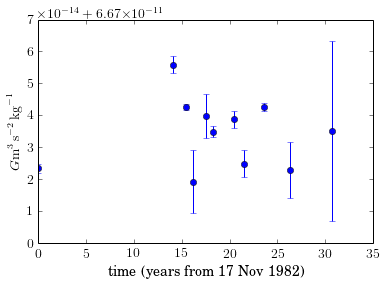In :
Lpost = np.zeros((len(sysrange), len(Arange), len(phi0range), len(periodrange)))
for i in range(len(sysrange)):
for j in range(len(Arange)):
for k in range(len(phi0range)):
for l in range(len(periodrange)):
model = pmodel(Arange[j], periodrange[l], phi0range[k], 0., years)
Lpost[i,j,k,l] = logL(Gsshuf, model, errshuf, sysrange[i])
Lpost[i,j,k,l] = Lpost[i,j,k,l] + logpriorsys(sysrange[i]) + logpriorA(Arange[j]) + logpriorphi0 + logpriorperiod

# marginalise over parameters
marg1 = np.apply_along_axis(logtrapz, 3, Lpost, np.diff(periodrange))
marg2 = np.apply_along_axis(logtrapz, 2, marg1, np.diff(phi0range))
marg3 = np.apply_along_axis(logtrapz, 1, marg2, np.diff(Arange))

Zshuf = logtrapz(marg3, np.diff(sysrange))
print Zshuf

# plot posteriors
f, ax = pl.subplots(2, 2, figsize=(10,10))

# plot amplitude posterior
marg1 = np.apply_along_axis(logtrapz, 3, Lpost, np.diff(periodrange))
marg2 = np.apply_along_axis(logtrapz, 2, marg1, np.diff(phi0range))
marg3 = np.apply_along_axis(logtrapz, 0, marg2, np.diff(sysrange))

ax[1,1].plot(Arange, np.exp(marg3-Zshuf))
ax[1,1].set_xlabel('$A (\mathrm{m}^3\,\mathrm{s}^{-2}\,\mathrm{kg}^{-1})$')
ax[1,1].set_ylabel('$p(A|d,H,I)$')

# plot period posterior
marg1 = np.apply_along_axis(logtrapz, 2, Lpost, np.diff(Arange))
marg2 = np.apply_along_axis(logtrapz, 1, marg1, np.diff(phi0range))
marg3 = np.apply_along_axis(logtrapz, 0, marg2, np.diff(sysrange))

ax[0,0].plot(periodrange, np.exp(marg3-Zshuf))
ax[0,0].set_xlabel('$P$ (years)')
ax[0,0].set_ylabel('$p(P|d,H,I)$')
ax[0,0].set_xlim(periodrange, periodrange[-1])

# get marginal distribution for period and amplitude
marg1 = np.apply_along_axis(logtrapz, 2, Lpost, np.diff(phi0range))
marg2 = np.apply_along_axis(logtrapz, 0, marg1, np.diff(sysrange))

post2d = np.exp(marg2-Zshuf)
ax[1,0].imshow(np.flipud(post2d), extent=[periodrange, periodrange[-1], Arange, Arange[-1]], aspect='auto')
ax[1,0].set_xlabel('$P$ (years)')
ax[1,0].set_ylabel('$A (\mathrm{m}^3\,\mathrm{s}^{-2}\,\mathrm{kg}^{-1})$')

ax[0, 1].axis('off')

335.648889857


Out:
(0.0, 1.0, 0.0, 1.0)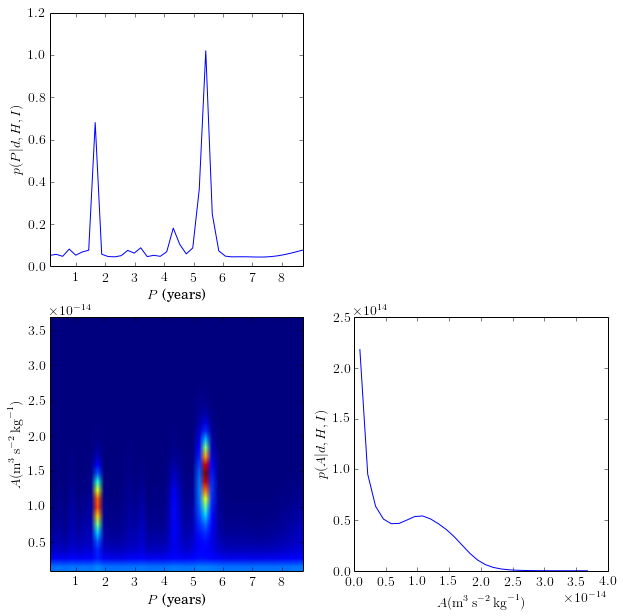This just goes to show that finding a periodic component like the suggested one that Anderson et al, and observed as a spike with a period near 5.9 years in my period posterior for hypothesis 5, in not a significant thing. It can essentially be reproduced with data that is just noise.